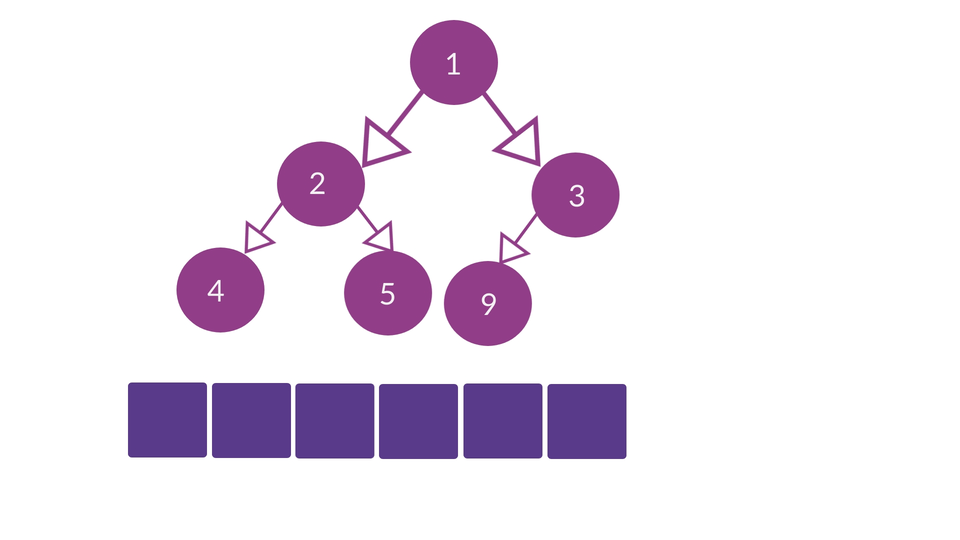# Heap Data Structure using Arrays

Written by Richa Kiran on Jan 8th, 2022 Views

A heap is a Complete Binary Tree in which elements are arranged in a specific order depending the type of Heap that we're trying to implement. It can be either the first element(root node) being the smallest followed by bigger elements in the following levels(Min Heap) or first element being the largest, followed by smaller elements in the following levels(Max Heap). So far, we've seen how we can implement heaps with binary tree data structure and what are the possible disadvantages maybe associated with that method. In this article, we're gonna see how it's implemented using arrays.

## The Algorithm

To be able to represent a heap in the form of an array, we have some formulae to determine which element of the heap can be inserted at a given index in an array. Following are some simple points to remember while converting a heap into an array -

1. The root node is always going to be at index 0 of the array.
2. if "i" is an index of the array then -
1. Left child of a node will be placed at: 2*i+1
2. Right child of a node will be placed at: 2*i+2
3. Parent node will be at: (i-1)/2

Overall, the representation of a Min Heap in the form of an array looks something like this -So, all you have to do is -

1. Make an array of n size, n being the number of elements in the heap.
2. Make a variable i, ranging between 0 to n-1.
3. i=0 is the starting value of i, which represents the root node of the heap. So, at i=0, insert the root element.
4. Now, by using the given formulae, insert the left and right child of the root node.
5. Increment the value of i by 1.
6. Repeat steps 3-5 for i'th node. If for some i'th node, either of the child nodes do not exist then end the process.
7. The resulting array is the array representation of the heap.

Note that the heaps that you use for this process is heapified, which means that it already contains the elements in the sorted order(like the heap we used in the animation).

## Conclusion

There are many other operations that you can perform on the array equivalent of heaps some of them being - insertion, deletion and heapify(to sort an unsorted array). In my upcoming articles I'll be covering such operations. I hope this article gave you a clear understanding of how we can generate and array of elements present in a heap.

To read more such articles, you can visit my page here.